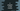# C program to find the median of an array## C program to find the median of an array:

In this post, we will learn how to find the median of an array in C. The median value is the value at the middle of a sorted array. To calculate the median, we need to sort the array first in ascending or descending order and then we can pick the element at the center.

The program will take the value of n as an input from the user, then it will take the numbers of the array and finally print the median value.

### Algorithm to use:

Before we move to the final program, let’s learn the algorithm first:

• The median of an array is the middle value of a sorted array.
• If the array includes even number of elements, then the median is the average of the two numbers in the middle of the array.
• If the array includes odd number of elements, then the middle number of the array is the median.
• The first thing we need to do is to sort the array before we calculate the median value. You can use any sorting algorithm to sort it. In this example, we will use bubble sort.
• Once the array is sorted, based on its length, the program will find the median value and print it.

Let’s write down the program.

### C program to find the median of an array:

Below is the complete C program that finds the median of a user input array:

``````#include <stdio.h>

void sort(int *arr, int size)
{
int i, j, t;

for (i = 0; i < size; i++)
{
for (j = i + 1; j < size; j++)
{
if (arr[j] < arr[i])
{
t = arr[i];
arr[i] = arr[j];
arr[j] = t;
}
}
}
}

float getMedian(int *arr, int size)
{
if (size % 2 == 0)
{
return (arr[(size - 1) / 2] + arr[size / 2]) / 2.0;
}
else
{
return arr[size / 2];
}
}

int main()
{
int i, size;

printf("Enter the total number of elements: ");
scanf("%d", &size);

int arr[size];

for (i = 0; i < size; i++)
{
printf("Enter the element for index %d: ", i);
scanf("%d", &arr[i]);
}

sort(arr, size);

printf("Median of the given array: %.2f\n", getMedian(arr, size));

return 0;
}``````

Here,

• The main() method runs first. The i and size are integer variables to use in the loop and to hold the size of the array.
• It reads the size of the array and create an array equal to that size. We are creating the array after the size of the array is entered by the user. This way, we can create an array of the exact size the user entered.
• The for loop reads the elements of the array one by one and stores these in that array.
• The sort method uses bubble sort to sort an array and getMedian returns the median value.
• The return value of getMedian is a floating point value. The last line is printing this value to the user.

### Output:

If you run the above program, it will print output as like below:

``````Enter the total number of elements: 7
Enter the element for index 0: 1
Enter the element for index 1: 2
Enter the element for index 2: 3
Enter the element for index 3: 5
Enter the element for index 4: 6
Enter the element for index 5: 9
Enter the element for index 6: 4
Median of the given array: 4.00

Enter the total number of elements: 4
Enter the element for index 0: 10
Enter the element for index 1: 5
Enter the element for index 2: 8
Enter the element for index 3: 2
Median of the given array: 6.50``````

For the first example, the following are the entered numbers: 1, 2, 3, 5, 6, 9, 4. If we sort this array, it will be 1, 2, 3, 4, 5, 6, 9. The size of the array is 7, which is odd. So, the fourth element of the sorted array is the median.

For the second example, entered numbers are 10, 5, 8, 2. If we sort this array, it will be 2, 5, 8, 10. The size of the array is 4. So, the median is the average of the second and the third number i.e. (5 + 8)/2, or 13/2 = 6.5.

This program will work with any number of elements. You can try with different size arrays and it will give the median.